Physicists Biographies

# vector decomposition

When we refer to scalar quantities, we do not need to use a specific word to express its measurement, it is enough to express the measurement itself, for example: the mass of a body is 2 kg, the length of the track is 5 km, etc. . But when we are working with vector quantities , this procedure is no longer correct, as the expression of the measure only gives a part of the information, for example: saying that the force applied to a body is 4 N does not indicate the direction and direction of the force.

For this reason, in vector quantities, it is correct to say: the magnitude of the force applied to a body is 4 N. In other situations that deal with vector quantities, we have to add or subtract vectors in the same direction. A very interesting case that deserves a lot of attention is the sum of vector quantities with vectors arranged perpendicularly to each other.

When you come across a situation where you need to add or subtract vectors perpendicular to each other, the best thing you can do is work with vector decomposition .

In the sum of two vectors, we can find only a single vector, that is, the resulting vector, which is nothing more than a vector that is equivalent to these two vectors. In vector decomposition, the process is reversed. Given a vector, we can find two other vectors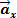and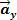such that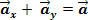Let’s see the figure below:

In this case, asandare vectors perpendicular to each other, the decomposition is orthogonal. See the figure below:

we can move the vectorto the end of the vectorso that the vector   andits orthogonal component vectors  form a right triangle.Based on the trigonometric relation applied to a right triangle, we can determine the magnitude of the horizontal and vertical components of the vectoras a function of the angle θ. So, from the yellow triangle above we have:

The expression of the horizontal component module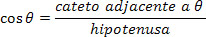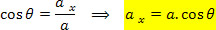The expression of the vertical component module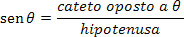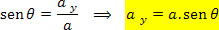Finally, as the triangle formed byand its components is a right triangle, applying the Pythagorean theorem, we have: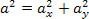which is the relationship between the magnitude of the vector and the magnitude of its orthogonal components.